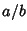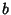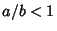## Fraction

A Rational Number expressed in the form, whereis called the Numerator andis called the Denominator. A Proper Fraction is a fraction such that, and a Lowest Terms Fraction is a fraction with common terms canceled out of the Numerator and Denominator.

The Egyptians expressed their fractions as sums (and differences) of Unit Fractions. Conway and Guy (1999) give a table of Roman Notation for fractions, in which multiples of 1/12 (the Uncia) were given separate names.

See also Adjacent Fraction, Anomalous Cancellation, Continued Fraction, Denominator, Egyptian Fraction, Farey Sequence, Golden Rule, Half, Lowest Terms Fraction, Mediant, Numerator, Proper Fraction, Pythagorean Fraction, Quarter, Rational Number, Unit Fraction

References

Conway, J. H. and Guy, R. K. The Book of Numbers. New York: Springer-Verlag, pp. 22-23, 1996.

Courant, R. and Robbins, H. ``Decimal Fractions. Infinite Decimals.'' §2.2.2 in What is Mathematics?: An Elementary Approach to Ideas and Methods, 2nd ed. Oxford, England: Oxford University Press, pp. 61-63, 1996.